# Fault diagnosis of ship power station based on LSTM neural network algorithm

## Shuheng Wang1, Yu Zhao2, Ning Chen3

1, 2, 3School of Energy and Power, Jiangsu University of Science and Technology, Zhenjiang, China

1Corresponding author

Vibroengineering PROCEDIA, Vol. 41, 2022, p. 204-210. https://doi.org/10.21595/vp.2022.22516
Received 15 March 2022; received in revised form 29 March 2022; accepted 8 April 2022; published 21 April 2022

55th International Conference on Vibroengineering, Almaty, Kazakhstan, April 21, 2022

Copyright © 2022 Shuheng Wang, et al. This is an open access article distributed under the Creative Commons Attribution License, which permits unrestricted use, distribution, and reproduction in any medium, provided the original work is properly cited.
Views 44
CrossRef Citations 0
Abstract.

Ship power station is an important part of the ship, and the fault types of ship power station are complex. In this paper, Simulink software platform is used to simulate and model various short-circuit faults of ship power station. The current and voltage parameters of each phase are selected as the source of the data set, and the data are processed and the prediction image is plotted in MATLAB. LSTM neural network algorithm solves the problem of long-term dependence compared with other algorithms, and has a high degree of interpretation of the predicted data. The results show that the fault diagnosis model based on LSTM neural network algorithm can well diagnose the fault mode of ship power station.

Highlights
• In this paper, MATLAB & Simulink software platform is used for dynamic simulation and short-circuit fault simulation of the operation of the ship power station, and LSTM neural network algorithm is used to train and diagnose the simulation data.
• Compared with the traditional RNN network model, LSTM has the ability to save historical information and shows obvious advantages in the prediction of time series. It can better predict time series with the help of current value and historical data.
• Compared with BP neural network algorithm, LSTM has better prediction accuracy, faster prediction speed, stronger sample accommodation, and can deal with the classification and prediction of big data.

Keywords: ship power station, short circuit fault, Simulink, LSTM neural network, fault diagnosis.

#### 1. Introduction

With the development of world economy and science and technology, the demand for ships is increasing, and the requirements for ship power stations are increasing. The load types of ships are becoming more and more complex, and the number of generators required for ship power stations is also increasing . The ship power station achieves the condition of high power consumption by paralleling several generators. In practice, marine generators may have various problems, which will seriously affect the safe and stable navigation of ships. Only the stable operation of the ship power station can ensure the stable navigation of the ship. Therefore, the study of ship power station is of great significance.

At present, the fault diagnosis of ship power stations has been studied to varying degrees in China and abroad. King et al. realized intelligent fault diagnosis of generator set by analyzing generator set fault and combining BP neural network algorithm . Sun Qiudong et al. designed a generator fault diagnosis system based on fuzzy production rule reasoning by using SJY-1 radio frequency monitor and fuzzy reasoning method, and successfully identified the generator insulation fault .

With the development of intelligent and intelligent algorithms for ship power stations, the combination of fault diagnosis and intelligent algorithms for ship power stations will be more and more closely. Selecting appropriate intelligent algorithm to diagnose ship fault is of great significance to fault analysis of ship power station.

#### 2. Ship power station simulation model

Ship power station simulation model consists of three parts, data input part, ship power station simulation model and data output part. The data input part is the system start, system stop, fault setting command data, load switching command data, and the data output part is the generator operation state data .

This experiment only needs to accurately and timely judge the generator fault mode. Therefore, this experiment only studies the simulation of single machine model, and sets different faults through the fault module to analyze the current and voltage data. As shown in Fig. 1, the single machine simulation model consists of a generator model, excitation system, diesel engine and speed control system. The increase and decrease of the load are controlled by the circuit breaker module, and different faults are set by the fault setting module to simulate the operation of the ship power station under different fault conditions.

Fig. 1. Single machine model simulation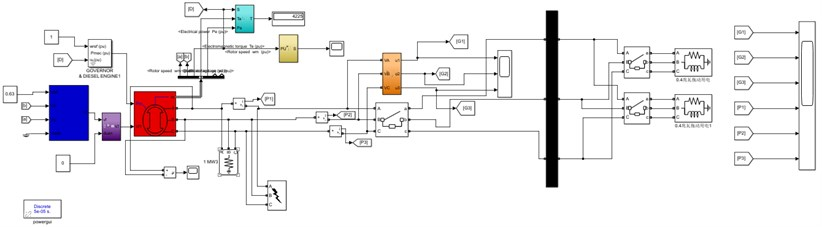#### 3.1. LSTM neural network

LSTM is the enhancement of ordinary cyclic neural network, which often causes the problem of gradient disappearance or gradient explosion . By changing the internal structure of the unit, the long-term and short-term memory network can avoid the above problems caused by the long time step, and can theoretically learn the data of any length of time step. The algorithm based on LSTM can realize language translation, robot control, speech recognition image recognition, handwritten text recognition, disease diagnosis and analysis, stock trend prediction, music composition and other tasks, which can well solve the long-term dependence problem. In industrial control, LSTM is mainly used for fault prediction and analysis .

#### 3.2. LSTM data set

Combined with the above mentioned ship generator fault simulation model, running generator stand-alone standard condition simulation model and fault condition simulation model . The Scope module in MATLAB is used to save the simulated data to the working area of MATLAB, and the values of each input parameter are viewed. The Scope model is added to the output position of the current and voltage parameters mentioned above, and six sets of data tables can be obtained by running the simulation model. The table is saved under the MATLAB working path as the input parameters of the following LSTM neural network training model.

#### 4.1. LSTM neural network initialization preparation

This paper uses code to establish LSTM neural network directly in MATLAB script file. Input the data set into LSTM neural network training model, take the first 90 % of the data for training, the last 10 % for testing. Parameter setting:  iteration number: 800;  Gradient threshold: 1;  initial learning rate: 0.006;  multiplier factor: 130;  Decrease factor: 0.3.

#### 4.2. Model training

Firstly, the three-phase current and voltage of the generator under normal and fault conditions are collected by simulating the four working conditions. The more stable 10000 sets of three-phase current and voltage data of generators are selected under each condition, and the four working conditions are represented by number 1-4 respectively. The number of specific sample data is selected as shown in Table 1.

After 800 iterations, the neural network completes the training and learning. After the completion of the training, the parameters are input into the chart, and the predicted monitoring value can be obtained by the algorithm model, and then the specific generator fault type can be judged .

Table 1. Fault type, sample number and fault number of ship power station

 Fault type Sample number Fault number Normal operation 10000 1 Single-phase earth fault 10000 2 Line-to-line grounded fault 10000 3 Three-phase short circuit 10000 4

#### 4.2.1. Normal operation

There is no short circuit fault in the normal operation of the generator set. The performance diagram of LSTM neural network training results is shown in Fig. 2. The comparison between the neural network prediction diagram and the original data observation diagram is shown in Fig. 3.

Fig. 2. Performance diagram of training results under normal conditions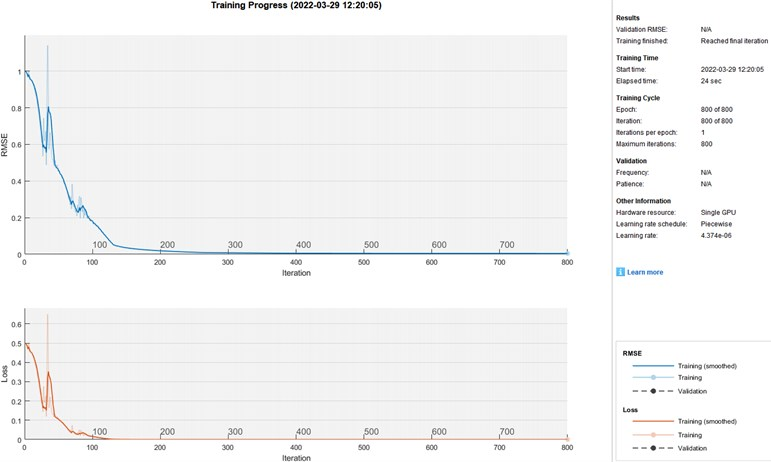Fig. 3. Comparison chart of normal working condition prediction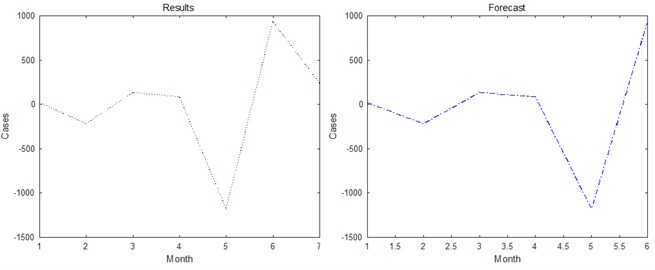#### 4.2.2. Single-phase earth fault

By setting a phase grounding short circuit through the fault module, the performance diagram of LSTM neural network training results is shown in Fig. 4. The comparison between the neural network prediction diagram and the original data observation diagram is shown in Fig. 5.

Fig. 4. Performance diagram of single-phase grounding short circuit training results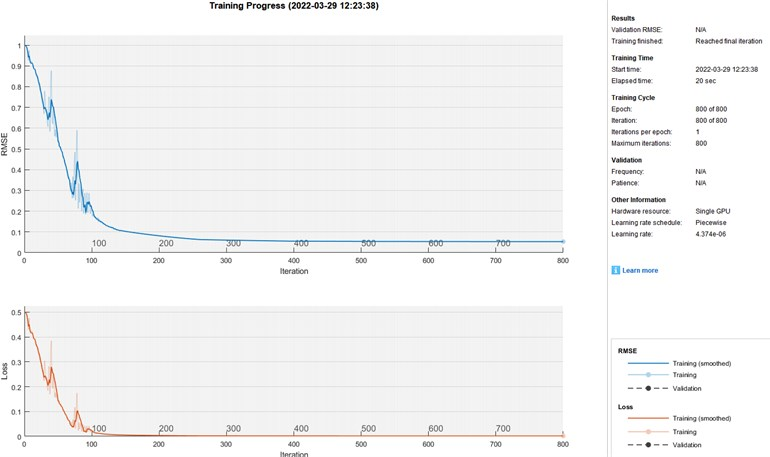Fig. 5. Comparison chart of single-phase grounding short circuit prediction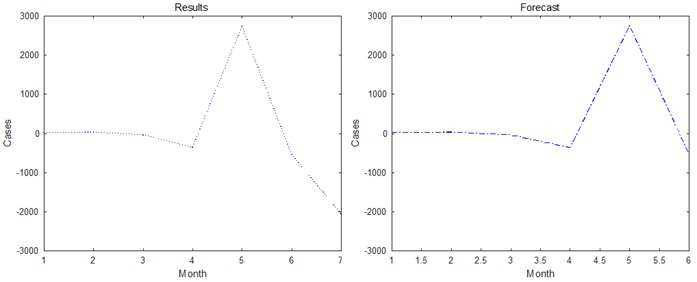#### 4.2.3. Line-to-line grounded fault

The phase-to-phase short circuit between a and b is set through the fault module. The performance diagram of LSTM neural network training results is shown in Fig. 6. The comparison between the neural network prediction diagram and the original data observation diagram is shown in Fig. 7.

#### 4.2.4. Three-phase short circuit

The a, b, c three-phase short circuit is set through the fault module, and the performance diagram of LSTM neural network training results is shown in Fig. 8. The comparison between the neural network prediction diagram and the original data observation diagram is shown in Fig. 9.

Fig. 6. Performance diagram of two-phase short circuit training results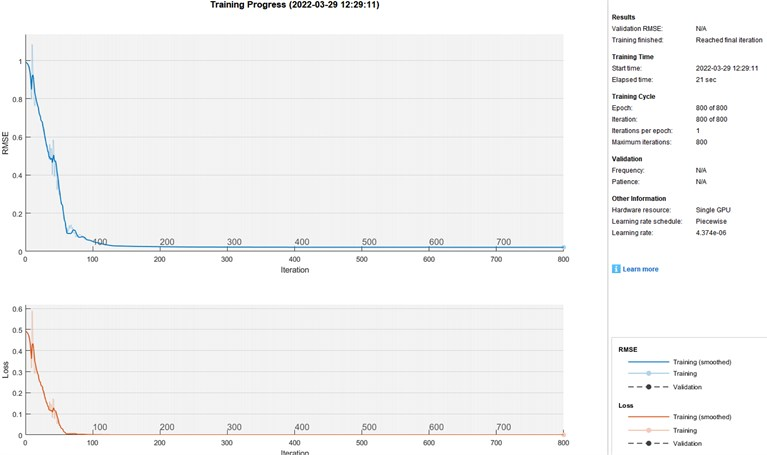Fig. 7. Comparison chart of two-phase short circuit prediction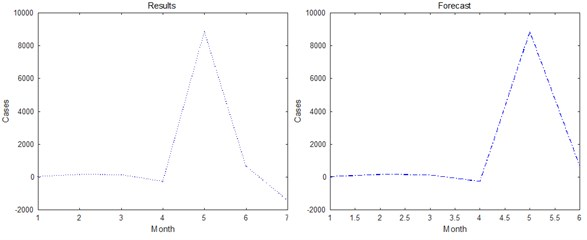Fig. 8. Performance diagram of three phase short circuit training results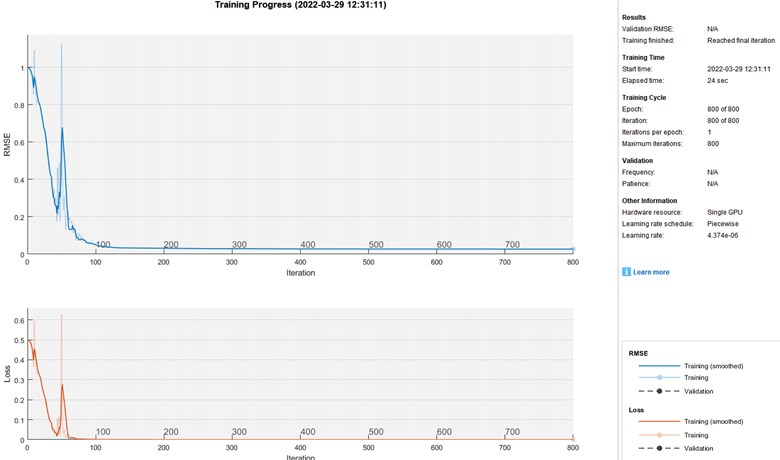Fig. 9. Comparison chart of three-phase short circuit prediction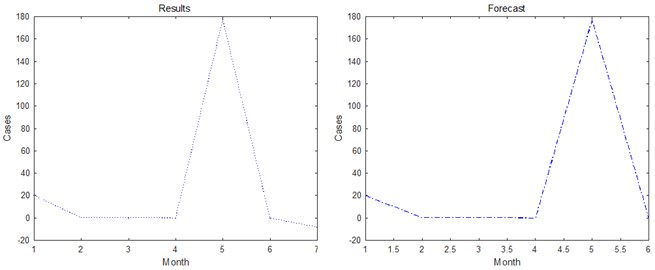#### 4.3. Comparison between LSTM and BP neural network

The BP neural network module in Simulink is connected to the power station model. The collected current and voltage parameters are connected to the BP neural network module as the input end, and each working condition is operated to obtain the prediction results of the BP neural network. Compared with the prediction parameters obtained by LSTM neural network, the comparison data are shown in Table 2.

Table 2. LSTM and BP neural network fault diagnosis model data comparison

 Diagnosis model Diagnostic accuracy Diagnosis time LSTM neural network 99.986 % 15.902 s BP neural network 96.024 % 47.325 s

#### 4.4. Experimental conclusion

The above pictures show that the LSTM neural network prediction maps are quite different under different fault conditions, and the generator short-circuit fault can be accurately judged. The fitting degree of training results and input values is also very high. And the training results show that the root mean square error is small, indicating that the variable has a strong ability to explain the predicted value. Compared with BP neural network, LSTM neural network has faster fault diagnosis time and higher accuracy. The experimental results show that LSTM neural network can be well used in fault diagnosis of ship power station.

#### 5. Conclusions

This paper introduces a fault diagnosis model of ship power station based on LSTM neural network algorithm. The generator simulation model is built based on the Simulink platform in MATLAB. On this basis, the model is simulated for different faults, and the data set required by the neural network algorithm is collected. In terms of algorithm, the long-term and short-term memory network is selected. Compared with other neural networks, LSTM network can realize the long-term memory of information, and has better explanatory power for the predicted value. The algorithm code is established in MATLAB platform, the data set is substituted, and the diagnosis results are obtained by running the model. Through the final results, LSTM neural network can well complete the fault diagnosis of ship power station.

1. G. Erceg, S. Tešnjak, and R. Erceg, “Modeling and simulation of diesel electrical aggregate,” Proceedings of KOREMA'96 – 41st Annual Conference, Vol. 10, No. 2, pp. 49–52, 1996. [Search CrossRef]
2. Y. Zhang, “Marine generator common faults and intelligent fault diagnosis methods,” Modern Industrial Economy and Informationization, Vol. 22, No. 6, pp. 219–224, 2016. [Search CrossRef]
3. H. He, H. Li, and J. Shen, “Modeling and simulation of converter unit on ship,” Computer Simulation, Vol. 24, No. 6, pp. 229–234, 2007. [Search CrossRef]
4. Z. Yin, H. Zhao, and Y. Yuan, “Design and research of marine generator intelligent diagnosis system,” Ship Science and Technology, Vol. 32, No. 2, pp. 41–44, 2016. [Search CrossRef]
5. Y. Yu, X. Si, C. Hu, and J. Zhang, “A review of recurrent neural networks: LSTM cells and network architectures,” Neural Computation, Vol. 31, No. 7, pp. 1235–1270, Jul. 2019, https://doi.org/10.1162/neco_a_01199 [Publisher]
6. Z. Zhong, J. Yan, W. Wu, J. Shao, and C.-L. Liu, “Practical block-wise neural network architecture generation,” in 2018 IEEE/CVF Conference on Computer Vision and Pattern Recognition (CVPR), pp. 2423–2432, Jun. 2018, https://doi.org/10.1109/cvpr.2018.00257 [Publisher]
7. P. Cheng, J. Li, and H. Lan, “Modeling and PSCAD simulation analysis on a ship power system,” in Applied Mechanics and Materials, pp. 58–62, 2012. [Publisher]
8. L.-K. Song, G.-C. Bai, and C.-W. Fei, “Multi-failure probabilistic design for turbine bladed disks using neural network regression with distributed collaborative strategy,” Aerospace Science and Technology, Vol. 92, pp. 464–477, Sep. 2019, https://doi.org/10.1016/j.ast.2019.06.026 [Publisher]